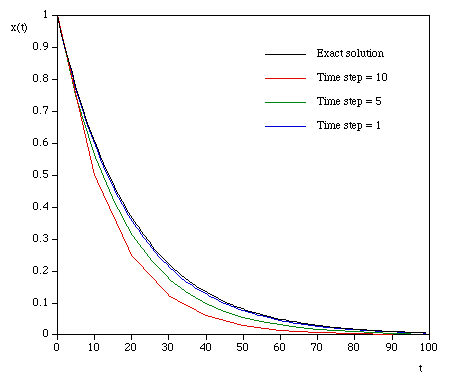# Solving systems defined by differential equations

Written by Paul Bourke
January 1997

Differential equations often arise in modelling and simulation exercises. A differential equation defines the relationship between an unknown function and its derivative, numerical methods are required to find the function defined by the differential equation(s).

This document will describe some standard methods for solving what are known as ordinary differential equations (ODE) of the form:

dy / dt = f(y)

This generally describes functions of a single variable (y). While the derivative is with respect to time (t), it is a straightforward change for other variables. The solution for higher derivatives is also possible as they can be changed to a number of systems with only first order derivatives.

The simplest method of solving such equations is known as the Euler method, the estimate of the next value yi+1 given the current value yi is

yi+1 = yi + h f(yi)

Note this is a discrete series approximation to the "real" function, the time between each time step is h. One key feature of different algorithms is how small the step size needs to be between each estimate of the function so that the solution is stable and beneath some error. The smaller the step size the better the estimate but the longer it takes, this is particularly so for computationally expensive derivative functions. The Euler method needs the smallest number of calculations of the derivative per time step (1) but it also needs the smallest step size for a given error bound.

The basis of the Euler method and the other possible algorithms is based on the Taylor series expansion

y(t + h) = y(t) + h y'(t) + h2 y''(t) / 2! + h3 y'''(t) / 3! + ..... + hn (dny / dtn) / n!

The Euler method is just the first two terms of the expansion, the error term is order h2, O(h2).

An improved algorithm which involves estimating the second derivative is called the midpoint method and is given by

yi+1 = yi + h f(yi + h f(yi) / 2)

The error of the midpoint method is O(h3). For the same error it is possible to increase the step size, the price is that we need to evaluate the derivative function twice per step.

Other algorithms such as the Runge-Kutta do even better, if we look at the most common 4'th order version it evaluates the derivative function 4 times and has an error of O(h5).

There are other algorithms which have adaptive step sizes, the permissible error is given and the step size is continuously adjusted to achieve less than the specified error. These techniques will not be discussed here.

The following C source provides precise details on a number of algorithms.

```/*
A 1st order 1D DE solver.
Assumes the function is not time dependent.
Parameters
h      - step size
y0     - last value
method - algorithm to use [0,5]
fcn    - evaluate the derivative of the field
*/
double Solver1D(double h,double y0,int method,double (*fcn)(double))
{
double ynew;
double k1,k2,k3,k4,k5,k6;

switch (method) {
case 0:                          /* Euler method */
k1 = h * (*fcn)(y0);
ynew = y0 + k1;
break;
case 1:                          /* Modified Euler */
k1 = h * (*fcn)(y0);
k2 = h * (*fcn)(y0 + k1);
ynew = y0 + (k1 + k2) / 2;
break;
case 2:                          /* Heuns method */
k1 = h * (*fcn)(y0);
k2 = h * (*fcn)(y0 + 2 * k1 / 3);
ynew = y0 + k1 / 4 + 3 * k2 / 4;
break;
case 3:                          /* Midpoint */
k1 = h * (*fcn)(y0);
k2 = h * (*fcn)(y0 + k1 / 2);
ynew = y0 + k2;
break;
case 4:                          /* 4'th order Runge-kutta */
k1 = h * (*fcn)(y0);
k2 = h * (*fcn)(y0 + k1/2);
k3 = h * (*fcn)(y0 + k2/2);
k4 = h * (*fcn)(y0 + k3);
ynew = y0 + k1 / 6 + k2 / 3 + k3 / 3 + k4 / 6;
break;
case 5:                          /* England 4'th order, six stage */
k1 = h * (*fcn)(y0);
k2 = h * (*fcn)(y0 + k1 / 2);
k3 = h * (*fcn)(y0 + (k1 + k2) / 4);
k4 = h * (*fcn)(y0 - k2 + 2 * k3);
k5 = h * (*fcn)(y0 + (7 * k1 + 10 * k2 + k4) / 27);
k6 = h * (*fcn)(y0 + (28*k1 - 125*k2 + 546*k3 + 54*k4 - 378*k5) / 625);
ynew = y0 + k1 / 6 + 4 * k3 / 6 + k4 / 6;
break;
}

return(ynew);
}
```
A simple C program that illustrates how the above might be called is
```#include "stdio.h"
#include "stdlib.h"
#include "math.h"

#define EULER    0
#define MIDPOINT 3

double EvalFcn(double);
double Solver1D(double,double,int,double (*)(double));

int main(int argc,char **argv)
{
double t;
double dt=0.1;    /* Step size            */
double T=100;     /* Simulation duration  */
double y = 1;     /* Initial value        */

for (t=0;t<T;t+=dt) {
printf("%g %g\n",t,y);
y = Solver1D(dt,y,MIDPOINT,(double (*)(double))EvalFcn);
}
}

/*
Sample derivative function
*/
double EvalFcn(double x)
{
return(-0.05 * x);
}
```
The derivative function above is particularly simple but because it has a simple solution it is suitable for testing and error analysis. The solution to

dx / dt = - k x

is

x(t) = e- k t

The following illustrates the error with the Euler method using various step sizes.